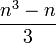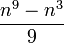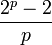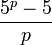# Formula for dimension of graded component of free Lie algebra

Jump to: navigation, search

## Statement

Suppose$n$ and$r$ are positive integers. Consider the free Lie algebra over$\mathbb{Q}$ with$n$ generators. Further, consider the degree$r$ graded component of this free Lie algebra. This is a$\mathbb{Q}$-vector space and is spanned by all the Lie brackets of length$r$ involving the generators. The dimension is equal to the number of equivalence classes of words of length$r$ in$n$ letters under cyclic permutations that have the property that no two cyclic permutations of the word are equal.

The expression is:$\frac{1}{r} \sum_{d \mid r} \mu(d) n^{r/d}$

where$\mu$ denotes the Mobius function.

Note that instead of using free$\mathbb{Q}$-Lie algebras, we could also have used the free$\mathbb{Z}$-Lie ring. In this case, it would turn out that each graded component is a free abelian group and the above formula gives the rank of that group.

## Particular cases

Each row corresponds to a value of$r$. For a given value of$r$, the dimension is a polynomial in$n$ of degree$r$. The polynomial is listed, then its values for small$n$ are given.

There are two ways that more detail could be provided. One is to provide the explicit Lyndon basis -- for more on that, see the basic Lie product page. The other is to provide explicit decompositions in terms of Schur functors. For more on that, see induction formula for Lie operad.

Value of$r$ Polynomial sending$n$ to dimension Dimension case$n = 1$ Dimension case$n = 2$ Dimension case$n = 3$ Dimension case$n = 4$ Dimension case$n = 5$
1$n$ 1 2 3 4 5
2$\frac{n^2 - n}{2}$ 0 1 3 6 10
3$\frac{n^3 - n}{3}$ 0 2 8 20 40
4$\frac{n^4 - n^2}{4}$ 0 3 18 60 150
5$\frac{n^5 - n}{5}$ 0 6 48 204 624
6$\frac{n^6 - n^3 - n^2 + n}{6}$ 0 9 116 670 2580
7$\frac{n^7 - n}{7}$ 0 18 312 2340 11160
8$\frac{n^8 - n^4}{8}$ 0 30 810 8160 48750
9$\frac{n^9 - n^3}{9}$ 0 56 2184 29120 217000
prime$p$$\frac{n^p - n}{p}$ 0$\frac{2^p - 2}{p}$$\frac{3^p - 3}{p}$$\frac{4^p - 4}{p}$$\frac{5^p - 5}{p}$
prime power$p^t$$\frac{n^{p^t} - n^{p^{t-1}}}{p^t}$ 0$\frac{2^{p^t} - 2^{p^{t-1}}}{p^t}$$\frac{3^{p^t} - 3^{p^{t-1}}}{p^t}$$\frac{4^{p^t} - 4^{p^{t-1}}}{p^t}$$\frac{5^{p^t} - 5^{p^{t-1}}}{p^t}$
product$p_1p_2$ of distinct primes$\frac{n^{p_1p_2} - n^{p_1} - n^{p_2} + n}{p_1p_2}$ 0$\frac{2^{p_1p_2} - 2^{p_1} - 2^{p_2} + 2}{p_1p_2}$$\frac{3^{p_1p_2} - 3^{p_1} - 3^{p_2} + 3}{p_1p_2}$$\frac{4^{p_1p_2} - 4^{p_1} - 4^{p_2} + 4}{p_1p_2}$$\frac{5^{p_1p_2} - 5^{p_1} - 5^{p_2} + 5}{p_1p_2}$

## Facts used

1. Basic Lie products form a freely generating set of graded component of free Lie algebra

## Proof

The reason this result holds is that it counts the number of basic Lie products, which are known to form a basis for the vector space by Fact (1).